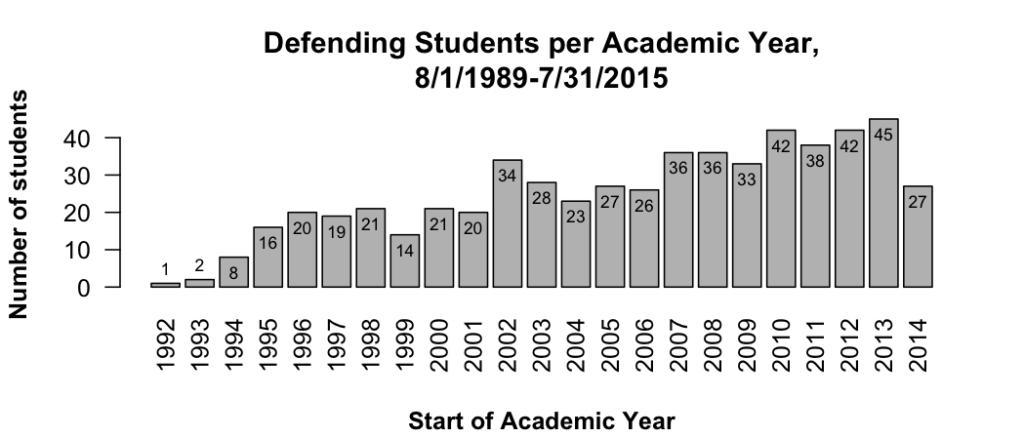Non-ggplot bar graph with data labels

p = function() {
x = gd\$year
y = gd\$defended_n
bp = barplot(
y,
names.arg = x,
las = 2 # make years vertical by making all ticks perpendicular
)
# title
# x axis
title(xlab="Start of Academic Year", font.lab=2, line=4)
# y axis
title(ylab="Number of students", font.lab=2)
# data data labels
label_height = y - 4
label_height = label_height + 8 # the first one is too low, bump it up
label_height = label_height + 8 # the second one is too low, bump it up
text(bp, label_height, y, cex=.7)
}Side-by-side bars with error bars

Simple side-by-side (no error bars):
http://stackoverflow.com/a/25070645

Hiding the legend

# Remove legend for a particular aesthetic (fill)
bp + guides(fill=FALSE)

# It can also be done when specifying the scale
bp + scale_fill_discrete(guide=FALSE)

# This removes all legends
bp + theme(legend.position="none")

Rotating Axis Labels

http://stackoverflow.com/questions/1330989/rotating-and-spacing-axis-labels-in-ggplot2

Also makes the text smaller (assuming you are rotating because of long labels).

q + theme(axis.text.x = element_text(angle = 90, hjust = 1, size=20))

Percent labels for axis

http://stackoverflow.com/questions/27433798/how-to-change-y-axis-range-to-percent-from-number-in-barplot-with-r

library(scales)
myplot <- qplot(as.factor(x), y, geom="bar") myplot + scale_y_continuous(labels=percent)

Dodging

Use position='dodge' when you are okay with the default behavior.

Use position=position_dodge(width = 0.90, height=2) when you want to control the behavior.

Zooming

If you use scale_y_XXX(limits=c(50, 100)), any graph object that falls outside that window at all will be completely removed from the figure, even if a different portion falls within the limits. To avoid this, use the following to 'zoom' in the figure, without changing what objects are included.

p + coord_cartesian(xlim = c(325, 500))

ggplot's text justification

http://stackoverflow.com/questions/7263849/what-do-hjust-and-vjust-do-when-making-a-plot-using-ggplot

Think of hjust as the percentage of the text's horizontal width that is left of the anchor point. So 0 means 0% of the text is left of the anchor point, so this is left justified. 0.5 means 50% of the text is left of the anchor point, so the text is right justified. 1 is 100% is right justified.

vjust is the percent of height **below** the anchor.

A way to remember this is that for an anchor point at 0,0, and with hjust=vjust=0, the text would appear properly in upper right quadrant from the origin.

Note that if the text is rotated, the justification is applied before the rotation. For example, text with hjust=0 and vjust=1 is right of and below the anchor point. If it is then rotated 90 degrees counterclockwise, it ends up right of but above the anchor point.

Axis label spacing

The default theme doesn't have enough space between axis text and tick marks.